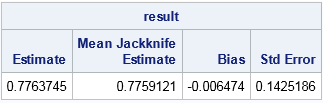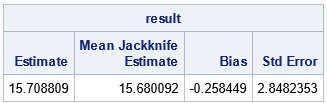One way to assess the precision of a statistic (a point estimate) is to compute the standard error, which is the standard deviation of the statistic's sampling distribution. A relatively large standard error indicates that the point estimate should be viewed with skepticism, either because the sample size is small or because the data themselves have a large variance. The jackknife method is one way to estimate the standard error of a statistic.

Some simple statistics have explicit formulas for the standard error, but the formulas often assume normality of the data or a very large sample size. When your data do not satisfy the assumptions or when no formula exists, you can use resampling techniques to estimate the standard error. Bootstrap resampling is one choice, and the jackknife method is another. Unlike the bootstrap, which uses random samples, the jackknife is a deterministic method.

This article explains the jackknife method and describes how to compute jackknife estimates in SAS/IML software. This is best when the statistic that you need is also implemented in SAS/IML. If the statistic is computed by a SAS procedure, you might prefer to download and use the %JACK macro, which does not require SAS/IML.

### The jackknife method: Leave one out!

The jackknife method estimates the standard error (and bias) of statistics without making any parametric assumptions about the population that generated the data. It uses only the sample data.

The jackknife method manufactures jackknife samples from the data. A jackknife sample is a "leave-one-out" resample of the data. If there are n observations, then there are n jackknife samples, each of size n-1. If the original data are {x1, x2,..., xn}, then the i_th jackknife sample is
{x1,..., xi-1,xi+1,..., xn}
You then compute n jackknife replicates. A jackknife replicate is the statistic of interest computed on a jackknife sample. You can obtain an estimate of the standard error from the variance of the jackknife replicates. The jackknife method is summarized by the following:

1. Compute a statistic, T, on the original sample of size n.
2. For i = 1 to n, repeat the following:
• Leave out the i_th observation to form the i_th jackknife sample.
• Compute the i_th jackknife replicate statistic, Ti, by computing the statistic on the i_th jacknife sample.
3. Compute the average (mean) of the jackknife replicates: Tavg = Σi Ti / n.
4. (Optional) Estimate the bias as BiasTjack = (n-1)(Tavg - T)
5. Estimate the standard error as SEjack = sqrt( (n-1)/n Σi (Ti - Tavg)**2 )

### Data for a jackknife example

Resampling methods are not hard, but the notation in some books can be confusing. To clarify the method, let's choose a particular statistic and look at example data. The following example is from Martinez and Martinez (2001, 1st Ed, p. 241), which is also the source for this article. The data are the LSAT scores and grade-point averages (GPAs) for 15 randomly chosen students who applied to law school.

```data law; input lsat gpa @@; datalines; 653 3.12 576 3.39 635 3.30 661 3.43 605 3.13 578 3.03 572 2.88 545 2.76 651 3.36 555 3.00 580 3.07 594 2.96 666 3.44 558 2.81 575 2.74 ;```

The statistic of interest (T) will be the correlation coefficient between the LSAT and the GPA variables for the n=15 observations. The observed correlation is TData = 0.776. The standard error of T helps us understand how much T would change if we took a different random sample of 15 students. The next sections show how to implement the jackknife analysis in the SAS/IML language.

### Construct a jackknife sample in SAS

The SAS/IML matrix language is the simplest way to perform a general jackknife estimates. If X is an n x p data matrix, you can obtain the i_th jackknife sample by excluding the i_th row of X. The following two helper functions encapsulate some of the computations. The SeqExclude function returns the index vector {1, 2, ..., i-1, i+1, ..., n}. The JackSample function returns the data matrix without the i_th row:

```proc iml; /* return the vector {1,2,...,i-1, i+1,...,n}, which excludes the scalar value i */ start SeqExclude(n,i); if i=1 then return 2:n; if i=n then return 1:n-1; return (1:i-1) || (i+1:n); finish;   /* return the i_th jackknife sample for (n x p) matrix X */ start JackSamp(X,i); return X[ SeqExclude(nrow(X), i), ]; /* return data without i_th row */ finish;```

### The jackknife method for multivariate data in SAS

By using the helper functions, you can carry out each step of the jackknife method. To make the method easy to modify for other statistics, I've written a function called EvalStat which computes the correlation coefficient. This function is called on the original data and on each jackknife sample.

```/* compute the statistic in this function */ start EvalStat(X); return corr(X)[2,1]; /* <== Example: return correlation between two variables */ finish;   /* read the data into a (n x 2) data matrix */ use law; read all var {"gpa" "lsat"} into X; close;   /* 1. compute statistic on observed data */ T = EvalStat(X);   /* 2. compute same statistic on each jackknife sample */ n = nrow(X); T_LOO = j(n,1,.); /* LOO = "Leave One Out" */ do i = 1 to n; Y = JackSamp(X,i); T_LOO[i] = EvalStat(Y); end;   /* 3. compute mean of the LOO statistics */ T_Avg = mean( T_LOO );   /* 4 & 5. compute jackknife estimates of bias and standard error */ biasJack = (n-1)*(T_Avg - T); stdErrJack = sqrt( (n-1)/n * ssq(T_LOO - T_Avg) ); result = T || T_Avg || biasJack || stdErrJack; print result[c={"Estimate" "Mean Jackknife Estimate" "Bias" "Std Error"}];```The output shows that the estimate of bias for the correlation coefficient is very small. The standard error of the correlation coefficient is estimated as 0.14, which is about 18% of the estimate.

To use this code yourself, simply modify the EvalStat function. The remainder of the program does not need to change.

### The jackknife method in SAS/IML: Univariate data

When the data are univariate, you can sometimes eliminate the loop that computes jackknife samples and evaluates the jackknife replicates. If X is column vector, you can computing the (n-1) x n matrix whose i_th column represents the i_th jackknife sample. (To prevent huge matrices, this method is best for n < 20000.) Because many statistical functions in SAS/IML operate on the columns of a matrix, you can often compute the jackknife replicates in a vectorized manner.

In the following program, the JackSampMat function returns the matrix of jackknife samples for univariate data. The function calls the REMOVE function in SAS/IML, which deletes specified elements of a matrix and returns the results in a row vector. The EvalStatMat function takes the matrix of jackknife samples and returns a row vector of statistics, one for each column. In this example, the function returns the sample standard deviation.

```/* If x is univariate, you can construct a matrix where each column contains a jackknife sample. Use for univariate column vector x when n < 20000 */ start JackSampMat(x); n = nrow(x); B = j(n-1, n,0); do i = 1 to n; B[,i] = remove(x, i)`; /* transpose to column vevtor */ end; return B; finish;   /* Input: matrix where each column of X is a bootstrap sample. Return a row vector of statistics, one for each column. */ start EvalStatMat(x); return std(x); /* <== Example: return std dev of each sample */ finish;```

Let's use these functions to get a jackknife estimate of the standard error for the statistic (the standard deviation). The data (from Martinez and Martinez, p. 246) have been studied by many researchers and represent the weight gain in grams for 10 rats who were fed a low-protein diet of cereal:

```x = {58,67,74,74,80,89,95,97,98,107}; /* Weight gain (g) for 10 rats */ n = nrow(x);   /* optional: visualize the matrix of jackknife samples */ *M = JackSampMat(x); *print M[c=("S1":"S10") r=("1":"9")];   /* Jackknife method for univariate data */ /* 1. compute observed statistic */ T = EvalStatMat(x); /* 2. compute same statistic on each jackknife sample */ T_LOO = EvalStatMat( JackSampMat(x) ); /* LOO = "Leave One Out" */ /* 3. compute mean of the LOO statistics */ T_Avg = mean( T_LOO` ); /* transpose T_LOO */ /* 4 & 5. compute jackknife estimates of bias and standard error */ biasJack = (n-1)*(T_Avg - T); stdErrJack = sqrt( (n-1)/n * ssq(T_LOO - T_Avg) ); result = T || T_Avg || biasJack || stdErrJack; print result[c={"Estimate" "Mean Jackknife Estimate" "Bias" "Std Error"}];```The output shows that the standard deviation of these data is about 15.7 grams. The jackknife method computes that the standard error for this statistic is about 2.9 grams, which is about 18% of the estimate.

In summary, jackknife estimates are straightforward to implement in SAS/IML. This article shows a general implementation that works for all data and a specialized implementation that works for univariate data. In both cases, you can adapt the code for your use by modifying the function that computes the statistic on a data set. This approach is useful and efficient when the statistic is implemented in SAS/IML.

ShareDistinguished Researcher in Computational Statistics

Rick Wicklin, PhD, is a distinguished researcher in computational statistics at SAS and is a principal developer of SAS/IML software. His areas of expertise include computational statistics, simulation, statistical graphics, and modern methods in statistical data analysis. Rick is author of the books Statistical Programming with SAS/IML Software and Simulating Data with SAS.

1.Hi dear still I am not clear about life table parameter data analysis, which have only single observation for each treatment
e.x
intrinsic rate of natural increase(rm) value ares t1=2.32,t2=2.25,t3=2.25,t4=2.31, so how do I get the ANOVA,and standard error of mean?

•I suggest you post your question to the SAS Support Communities. Include sample data and your SAS program.

2.Achintya Mengi on

Getting the below error while running the second code for Univariate data.

ERROR: (execution) Matrix has not been set to

•You can get help for SAS programming questions at the SAS Support Communities. You can post your code and the log.

In this case, the error is saying that a matrix has not been defined. I don't know which matrix, but the code assumes that the variable X contains the data, so check that.

3.Step 5: Estimate the standard error as SEjack = sqrt( (n-1)/n (Σi Ti - Tavg)**2 )

Should be:

SEjack = sqrt( (n-1)/n Σi(Ti - Tavg)**2 )

•You are correct. FIXED. Thanks.

4.For the jackknife method in SAS/IML: Univariate data, if n > 20000, what we should do? Thanks.

•In many (most?) situations, you shouldn't need jacknife statistics if the data are that large. Use an asymptotic result for the standard error, if it exists.

If you want to use the jacknife anyway, you can always use a loop, as shown for multivariate data. However, for efficiency, I'd break up the problem into sets of size n1, n2, ..., nk, where N=n1+n2+...+nk is the size of the sample. Then use matrices of size N x n_j to compute the jackknife statistics in a vectorized manner.

5.Thank you, Dr. Wicklin.

6.I think in the last example, n=15 is used, where it should be 10

•Yes, you are correct. I have updated to code and the output table to reflect the correct computation. Thank you!